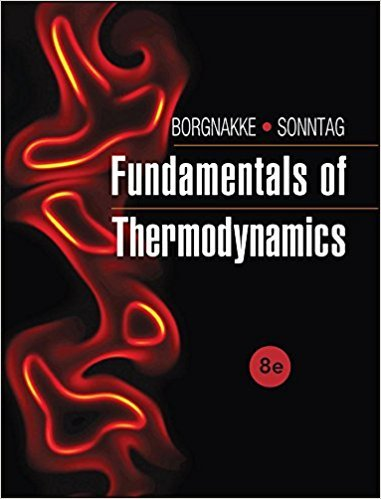×
Get Full Access to Fundamentals Of Thermodynamcs - 8 Edition - Chapter 4 - Problem 41hp
Get Full Access to Fundamentals Of Thermodynamcs - 8 Edition - Chapter 4 - Problem 41hp

×

# Turbines, ExpandersAir at 20 m/s, 1500 K, 875 kPa with 5ISBN: 9781118131992 58

## Solution for problem 41HP Chapter 4

Fundamentals of Thermodynamcs | 8th Edition

• Textbook Solutions
• 2901 Step-by-step solutions solved by professors and subject experts
• Get 24/7 help from StudySoup virtual teaching assistantsFundamentals of Thermodynamcs | 8th Edition

4 5 1 322 Reviews
30
0
Problem 41HP

Problem 41HP

Turbines, Expanders

Air at 20 m/s, 1500 K, 875 kPa with 5 kg/s flows into a turbine and it flows out at 25 m/s, 850 K, 105 kPa. Find the power output using constant specific heats.

Step-by-Step Solution:

Solution 41HP

Step 1 of 3:

Our aim is to find the power output of a turbine for the given data. Assume that specific heats in the process are constant.

The inlet mass flow rate= 5 kg/s

The inlet pressure P1 = 875 kPa

The inlet temperature T1 = 1500 K

The inlets velocity v1 = 20 m/s

The outlet pressure P2 = 105 kPa

The outlet temperature T2 = 850 K

The outlet velocity v2 = 25 m/s

The constant specific heat Cp0 = 1.004 kJ/kg.K (Table A.5)

Step 2 of 3

Step 3 of 3

##### ISBN: 9781118131992

This textbook survival guide was created for the textbook: Fundamentals of Thermodynamcs , edition: 8. The full step-by-step solution to problem: 41HP from chapter: 4 was answered by , our top Engineering and Tech solution expert on 08/03/17, 05:05AM. This full solution covers the following key subjects: kpa, flows, air, Find, constant. This expansive textbook survival guide covers 7 chapters, and 1462 solutions. Fundamentals of Thermodynamcs was written by and is associated to the ISBN: 9781118131992. The answer to “Turbines, ExpandersAir at 20 m/s, 1500 K, 875 kPa with 5 kg/s flows into a turbine and it flows out at 25 m/s, 850 K, 105 kPa. Find the power output using constant specific heats.” is broken down into a number of easy to follow steps, and 35 words. Since the solution to 41HP from 4 chapter was answered, more than 420 students have viewed the full step-by-step answer.

Unlock Textbook Solution